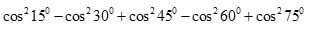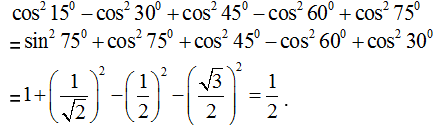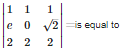# CBSE 12TH MATHEMATICS - Online Test

Q1.
The value ofis

Explaination / Solution:Q2.  is equal to
Explaination / Solution:

Q3. Order of a differential equation is defined as
Explaination / Solution:

Order of a differential equation is defined as the order of the highest order derivative of the dependent variable present in the differential equation.

Q4. If l, m, n are the direction cosines of a line, then
Explaination / Solution:

we know that direction cosines is coefficient of i,j,k of unit vector along that line ,i.e those coefficient are l,m,n .the length of the line r is such that  and magnitute of unit vector r is 1 and . on squaring both side we get
Q5. The area of the loop between the curve y = a sin x and the x – axis and x= 0 , x=π. is
Explaination / Solution:

Required area =
0πasinxdx=a[cosx]π0=a(cosπ+cos0)=a(1+1)=2a

Q6. The number of all possible matrices of order 3×3 with each entry 0 or 1 is
Explaination / Solution:

The number of elements in a 3 X 3 matrix is the product 3 X 3=9.

Each element can either be a 0 or a 1.

Given this, the total possible matrices that can be selected is 29=512

Q7. If the graph of a differentiable function y = f (x) meets the lines y = – 1 and y = 1, then the graph
Explaination / Solution:

Since the graph cuts the lines y = -1 and y = 1 , therefore ,it must cut y = 0 atleast once as the graph is a continuous curve in this case.

Q8.Explaination / Solution:because , row 1 and row 3 are identical.

Q9. Which of the following types of problems cannot be solved by linear programming methods
Explaination / Solution:

Traffic signal control types of problems cannot be solved by linear programming methods, because there is no need for optimization in such problems.

Q10. If R is a relation from a non – empty set A to a non – empty set B, then
Explaination / Solution:

Let A and B be two sets . Then a relation R from set A to set B is a subset of A×B.Thus , R is a relation from A to B ⇔R⊆A×B⇔R⊆A×B.